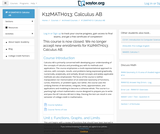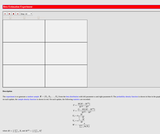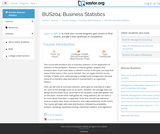# 194 Results

View
Selected filters:
• Probability and StatisticsConditional Remix & Share Permitted
CC BY-SA
Rating

This textbook is part of the OpenIntro Statistics series and offers complete coverage of the high school AP Statistics curriculum. Real data and plenty of inline examples and exercises make this an engaging and readable book. Links to lecture slides, video overviews, calculator tutorials, and video solutions to selected end of chapter exercises make this an ideal choice for any high school or Community College teacher. In fact, Portland Community College recently adopted this textbook for its Introductory Statistics course, and it estimates that this will save their students \$250,000 per year. Find out more at: openintro.org/ahss

View our video tutorials here:
openintro.org/casio
openintro.org/TI

Subject:
Probability and Statistics
Material Type:
Textbook
Provider:
OpenIntro
Author:
Christopher Barr
David Diez
Leah Dorazio
Mine Cetinkaya-Rundel
05/28/2019Unrestricted Use
CC BY
Rating

This course is oriented toward US high school students. The course is divided into 10 units of study. The first five units build the foundation of concepts, vocabulary, knowledge, and skills for success in the remainder of the course. In the final five units, we will take the plunge into the domain of inferential statistics, where we make statistical decisions based on the data that we have collected.

Subject:
Probability and Statistics
Material Type:
Full Course
Provider:
The Saylor Foundation
06/11/2019Conditional Remix & Share Permitted
CC BY-NC
Rating

Compare Histograms to stem-and-leaf, line plots and circle graphs.Mathematics Instructional Plans (MIPs) help teachers align instruction with the 2016 Mathematics Standards of Learning (SOL) by providing examples of how the knowledge, skills and processes found in the SOL and curriculum framework can be presented to students in the classroom.

Subject:
Mathematics
Probability and Statistics
Material Type:
Lesson Plan
Author:
Kristin Williams
Tina Mazzacane
04/29/2020Conditional Remix & Share Permitted
CC BY-NC
Rating

Collecting, organizing, representing, interpreting, and comparing data using line graphs Mathematics Instructional Plans (MIPs) help teachers align instruction with the 2016 Mathematics Standards of Learning (SOL) by providing examples of how the knowledge, skills and processes found in the SOL and curriculum framework can be presented to students in the classroom.

Subject:
Mathematics
Probability and Statistics
Material Type:
Lesson Plan
Author:
Doris Robinson
Debra Delozier
Tina Mazzacane
05/05/2020Conditional Remix & Share Permitted
CC BY-NC
Rating

Representing mean as the balance point Mathematics Instructional Plans (MIPs) help teachers align instruction with the 2016 Mathematics Standards of Learning (SOL) by providing examples of how the knowledge, skills and processes found in the SOL and curriculum framework can be presented to students in the classroom.

Subject:
Mathematics
Probability and Statistics
Material Type:
Lesson Plan
Author:
Doris Robinson
05/19/2020Unrestricted Use
CC BY
Rating

This resource consists of a Java applet and expository text. The applet is a simulation of the ballot experiment: The votes in an election are randomly counted. The event of interest is that the winning candidate is always ahead in the vote count.

Subject:
Probability and Statistics
Material Type:
Activity/Lab
Interactive
Simulation
Provider:
University of Alabama in Huntsville
Provider Set:
Virtual Laboratories in Probability and Statistics
Author:
Kyle Siegrist
05/04/2022Conditional Remix & Share Permitted
CC BY-NC
Rating

This lesson has students complete a basketball activity and then use the data from the activity to create a scatterplot. Once they create their scatterplot they determine correlation and draw a line of best fit.

Subject:
Probability and Statistics
Material Type:
Activity/Lab
Assessment
Author:
Sarah Leahey
01/05/2020Unrestricted Use
CC BY
Rating

This resource consists of a Java applet and expository text. The applet is a simulation of Bertrand's experiment: a random chord on a circle The event of interest is whether the length of the chord is larger than the length of the inscribed equilateral triangle. Three models for generating the random chord can be used.

Subject:
Probability and Statistics
Material Type:
Activity/Lab
Interactive
Simulation
Provider:
University of Alabama in Huntsville
Provider Set:
Virtual Laboratories in Probability and Statistics
Author:
Kyle Siegrist
05/04/2022Unrestricted Use
CC BY
Rating

This resource consists of a Java applet and expository text. The applet illustrates Bayesian estimation of the probability of heads for a coin. The prior beta distribution, true probability of heads, and the sample size can be specified. The applet shows the posterior beta distribution.

Subject:
Probability and Statistics
Material Type:
Activity/Lab
Interactive
Simulation
Provider:
University of Alabama in Huntsville
Provider Set:
Virtual Laboratories in Probability and Statistics
Author:
Kyle Siegrist
05/04/2022Unrestricted Use
CC BY
Rating

This resource consists of a Java applet and expository text. The applet simulates a random sample from a beta distribution, and computes standard point estimates of the left and right parameters. The bias and mean square error are also computed.

Subject:
Probability and Statistics
Material Type:
Activity/Lab
Interactive
Simulation
Provider:
University of Alabama in Huntsville
Provider Set:
Virtual Laboratories in Probability and Statistics
Author:
Kyle Siegrist
05/04/2022Unrestricted Use
CC BY
Rating

This resource consist of a Java applet and expository text. The applet simulates Bernoulli trials in terms of coin tosses. The random variables of interest are the number of heads and the proportion of heads. The number of coins and the probability of heads can be varied. The applet illustrates the law of large numbers and the central limit theorem.

Subject:
Probability and Statistics
Material Type:
Activity/Lab
Interactive
Simulation
Provider:
University of Alabama in Huntsville
Provider Set:
Virtual Laboratories in Probability and Statistics
Author:
Kyle Siegrist
05/04/2022Unrestricted Use
CC BY
Rating

This resource consists of a Java applet and expository text. The applet simulates Bernoulli trials in terms of random points on a timeline. The random variables of interest are the number of successes and the proportion of successes. The number of trials and the probability of success can be varied. This applet illustrates the law of large numbers, the central limit theorem, and the binomial distribution.

Subject:
Probability and Statistics
Material Type:
Activity/Lab
Interactive
Simulation
Provider:
University of Alabama in Huntsville
Provider Set:
Virtual Laboratories in Probability and Statistics
Author:
Kyle Siegrist
05/04/2022Unrestricted Use
CC BY
Rating

This resource consists of a Java applet and expository text. The applet is a simulation of the birthday experiment: a sample of size n is chose at random and with replacement from the first m positive integers. The random variable of interest is the number of distinct sample values. The event of interest is that all sample values are distinct.

Subject:
Probability and Statistics
Material Type:
Activity/Lab
Interactive
Simulation
Provider:
University of Alabama in Huntsville
Provider Set:
Virtual Laboratories in Probability and Statistics
Author:
Kyle Siegrist
05/04/2022Unrestricted Use
CC BY
Rating

This resource consists of a Java applet and expository text. The applet simulates the bivariate normal distribution. The means are set at 0, but the standard deviations and the correlation can be varied. Simulated points from the distribution are shown as dots in a scatterplot.

Subject:
Probability and Statistics
Material Type:
Activity/Lab
Interactive
Simulation
Provider:
University of Alabama in Huntsville
Provider Set:
Virtual Laboratories in Probability and Statistics
Author:
Kyle Siegrist
05/04/2022Unrestricted Use
CC BY
Rating

This resource consists of a Java applet and expository text. The Java applet illustrates the bivariate uniform distribution on three types of regions: a square, a circle, and a triangle. Simulated points from the distribution are shown as dots in a scatterplot.

Subject:
Probability and Statistics
Material Type:
Activity/Lab
Interactive
Simulation
Provider:
University of Alabama in Huntsville
Provider Set:
Virtual Laboratories in Probability and Statistics
Author:
Kyle Siegrist
05/04/2022Unrestricted Use
CC BY
Rating

This resource consists of a Java applet and expository text. The applet simulates Buffon's coin experiment. The radius of the coin can be varied. The applet illustrates a random experiment, the sample space, random variables, events, probability, and relative frequency.

Subject:
Probability and Statistics
Material Type:
Activity/Lab
Interactive
Simulation
Provider:
University of Alabama in Huntsville
Provider Set:
Virtual Laboratories in Probability and Statistics
Author:
Kyle Siegrist
05/04/2022Unrestricted Use
CC BY
Rating

This resource consists of a Java applet and expository text. The applet simulates Buffon's needle experiment and the corresponding approximation of pi. The event of interest is that the needle crosses a crack. The length of the needle can be varied. The applet illustrates a random experiment, the sample space, random variables, probability, and relative frequency.

Subject:
Probability and Statistics
Material Type:
Activity/Lab
Interactive
Simulation
Provider:
University of Alabama in Huntsville
Provider Set:
Virtual Laboratories in Probability and Statistics
Author:
Kyle Siegrist
05/04/2022Unrestricted Use
CC BY
Rating

Introductory survey of quantitative methods (QM), or the application of statistics in the workplace. Examines techniques for gathering, analyzing, and interpreting data in any number of fieldsĺÎĺ from anthropology to hedge fund management.

Subject:
Probability and Statistics
Material Type:
Activity/Lab
Full Course
Homework/Assignment
Syllabus
Provider:
The Saylor Foundation
06/11/2019Conditional Remix & Share Permitted
CC BY-NC-SA
Rating

Submitted as part of the California Learning Resource Network (CLRN) Phase 3 Digital Textbook Initiative (CA DTI3), CK-12 Advanced Probability and Statistics introduces students to basic topics in statistics and probability but finishes with the rigorous topics an advanced placement course requires. Includes visualizations of data, introduction to probability, discrete probability distribution, normal distribution, planning and conducting a study, sampling distributions, hypothesis testing, regression and correlation, Chi-Square, analysis of variance, and non-parametric statistics.

Subject:
Probability and Statistics
Material Type:
Textbook
Provider:
CK-12 Foundation
Provider Set:
CK-12 FlexBook
Author:
Almukkahal, Raja
09/14/2010Conditional Remix & Share Permitted
CC BY-NC-SA
Rating

CK-12 Middle School Math Concepts for seventh grade provides a complete textbook. It presents topics including algebraic thinking, patterns, decimals, decimal operations, fractions, fraction operations, integers, integer operations, ratios, rates, proportions, percents, percent applications, equations, solving equations, inequalities, functions, graphing functions, geometry, plane geometry, solid geometry, area, perimeter, surface area, volume, statistics including mean, median, mode and range, graphing and types of graphs, and probability.

Subject:
Mathematics
Algebra I & II
Measurement and Geometry
Probability and Statistics
Material Type:
Textbook
Provider:
CK-12 Foundation
Provider Set:
CK-12 FlexBook
Author:
Jen Kershaw